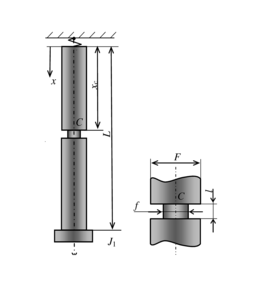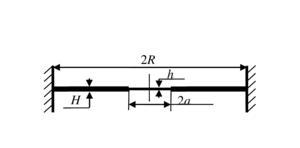ISSN 2658–5782
DOI 10.21662
Electronic Scientific Journalим. Р.Р. Мавлютова
УФИЦ РАНKhakimov A.G. Review of studies on the computational diagnosis of local defects of structural elements. Multiphase Systems. 14 (2019) 1. 1–9 (in Russian).
2019. Vol. 14. Issue 1, Pp. 1–9
URL: http://mfs.uimech.org/mfs2019.1.001,en
DOI: 10.21662/mfs2019.1.001
Review of studies on the computational diagnosis of local defects of structural elements
Khakimov A.G.
Mavlyutov Institute of Mechanics UFRC RAS, Ufa, Russia

### Abstract

Rods, beams, shafts, pipelines, round plates. membranes are parts of structures in which local defects such as cracks, dents, cavities, local corrosion can be formed. Provides an overview of the research A. G. Khakimov for the diagnosis of local defects. It is received: 1. The two eigenfrequencies of the longitudinal oscillations can determine the location and the notch parameter characterizing its size. 2. The three lower eigenfrequencies of the longitudinal oscillations can determine the initial coordinate and the value of the attached distributed mass to the stepped rod, as well as the ratio of the areas. 3. The solution of the inverse problem for extended rods allows us to determine the coordinate of the incision and the parameter containing its depth and length, according to the incident and reflected waves at the observation site. 4. The reflection from the air cavity and the passage of a longitudinal damped traveling wave in a pipeline immersed in a viscous liquid are determined, and the solution of the inverse problem allows to determine the coordinate of the air cavity and its length according to the reflected wave at the observation site. 5. The initial coordinate and the length of the longitudinal through radial section of the hollow shaft are determined by the two eigenfrequencies of torsional vibrations. 6. The coordinate, length and parameter of the drill string notch are determined by the three eigenfrequencies of the torsional vibrations. 7. The reflection from the distributed mass attached to the pipeline and the passage of the flexural traveling wave are considered. The dependence of the solution on the initial coordinate of the distributed mass and its magnitude is obtained, and the inverse problem of determining the initial coordinate of the distributed mass and its magnitude from the data of the reflected wave at the observation point is solved. 8. The radius and thickness of the thin central region of the membrane are determined by the two eigenfrequencies of the transverse vibrations. 9. The radius, thickness of the thinned central area of the plate and the value of the attached distributed mass are determined by the three natural frequencies of the bending oscillations. 10. By three frequencies of bending vibrations it is possible to determine the velocity parameter, the relative mass of the product per unit length of the pipeline and the relative mass of sediments on the walls of the pipeline and, as a consequence, the mass flow of liquid through the pipeline.

Keywords

rod,
shaft,
beam,
membrane,
plate,
pipeline,
defect,
computational diagnostics

## Article outline

Problem: Review of works devoted to the identification of defects in distributed mechanical systems using natural oscillation frequencies and transmitted and reflected waves.

Methods: Eigenfrequencies are used to detect a defect in a finite length rod and its location. In the case of rods of large length in comparison with the wavelength, this method of determining defects is inconvenient. More appropriate is the method of determining defects by transmission and reflection of waves. Identification of these defects is made by means of natural frequencies of oscillations, and also by means of passing and reflected waves. Local defects are modeled by coupling conditions, and cavities and cracks are modeled by continuous sections of the rod with altered stiffness, cross-sectional area and density.

In a study was determined:

1. The two eigenfrequencies of the longitudinal oscillations can determine the location and the notch parameter characterizing its size.

2. The three lower eigenfrequencies of the longitudinal oscillations can determine the initial coordinate and the value of the attached distributed mass to the stepped rod, as well as the ratio of the areas.

3. The solution of the inverse problem for extended rods allows us to determine the coordinate of the incision and the parameter containing its depth and length, according to the incident and reflected waves at the observation site.

4. The reflection from the air cavity and the passage of a longitudinal damped traveling wave in a pipeline immersed in a viscous liquid are determined. The solution of the inverse problem allows to determine the coordinate of the air cavity and its length according to the reflected wave at the observation site.

5. The initial coordinate and the length of the longitudinal through radial section of the hollow shaft are determined by the two eigenfrequencies of torsional vibrations.

6. The coordinate, length and parameter of the drill string notch are determined by the three eigenfrequencies of the torsional vibrations.

7. The stiffness parameter, the polar moment of inertia of the shaft in the second section, the initial coordinate and the length of this section can be determined by the four frequencies of free torsional vibrations.

8. The reflection and passage of a torsional wave propagating along an infinite rod with an artificial defect are considered. The solution of the inverse problem allows us to determine the coordinate of the rod damage and the parameter containing the depth and length of the defect by the characteristics of the incident and reflected waves at the observation site.

9. The reflection from the distributed mass attached to the pipeline and the passage of the bending traveling wave are considered. The dependence of the solution on the initial coordinate of the distributed mass and its magnitude is obtained, and the inverse problem of determining the initial coordinate of the distributed mass and its magnitude from the data of the reflected wave at the observation point is solved.

10. The radius and thickness of the thin Central region of the membrane are determined by the two eigenfrequencies of the transverse vibrations.

11. The radius, thickness of the thinned Central area of the plate and the value of the attached distributed mass are determined by the three natural frequencies of the bending oscillations.

12. By three frequencies of bending vibrations it is possible to determine the velocity parameter, the relative mass of the product per unit length of the pipeline and the relative mass of sediments on the walls of the pipeline and, as a consequence, the mass flow of liquid through the pipeline. The obtained results can be used for the acoustic method of pipeline diagnostics.

## References

1. Gladwell G.M.L. Inverse Problems in Vibration. 2nd ed. Dordrecht, Boston, London: Kluwer Academic Publishers, 2004.
2. Chu M.T., Golub G.H. Inverse Eigenvalue Problems: Theory, Algorithms, and Applications. Oxford University Press, USA, 2005. 406 p.
3. Axtyamov A. M. [Diagnostirovanie nagruzhenosti mexanicheskoj sistemy] Pribory i sistemy. Upravlenie, kontrol’, diagnostika [Devices and systems. Management, control, diagnostics]. 2003. No. 6. Pp. 60 (in Russian)
https://elibrary.ru/item.asp?id=23192890
4. Il’gamov M.A. [Diagnostics of damages of a vertical rod] Trudy Instituta mexaniki Ufimskogo nauchnogo centra RAN [Proceedings of the Mavlyutov Institute of Mechanics] V. 5. Ufa: Gilem. 2007. Pp. 201–211 (in Russian)
DOI: 10.21662/uim2007.1.025
5. Khakimov A.G. Damage Diagnostics in a Vertical Rod with Concentrated Masses on the Elastic Suspender // International Journal of Mechanics and Applications. 2012. V. 2, No.5, P. 70–73.
DOI: 10.5923/j.mechanics.20120205.03
6. Xakimov A.G. [On natural longitudinaql vibrations of a stepped rod having distributed attached mass] Kontrol’. Diagnostika [Control. Diagnostics]. 2013. No. 11. Pp. 9–13 (in Russian)
https://elibrary.ru/item.asp?id=20519086
7. Il’gamov M.A., Xakimov A.G.Reflection of a decaying traveling wave from a notch in a bar. Mechanics of Solids. 2011. No. 4. Pp. 589–596.
DOI: 10.3103/S0025654411040091
8. Il’gamov M.A., Xakimov A.G. [Reflection of a longitudinal wave from a notch in the rod immersed into a viscous fluid]. Vychislitel’naya mexanika sploshnyx sred. [Computational continuum mechanics]. 2010. V. 3. No. 3. Pp. 58–67 (in Russian).
DOI: 10.7242/1999-6691/2010.3.3.27
9. Il’gamov M.A., Xakimov A.G. [Reflection of Longitudinal Running Wave in Rod with Damages]. Kontrol’. Diagnostika. [Control. Diagnostics]. 2009. No. 7. Pp. 43–48 (in Russian).
https://elibrary.ru/item.asp?id=12882467
10. Il’gamov M.A., Xakimov A.G. [Longitudinal wave reflection from air cavity in a pipe system ]. Izvestiya Ufimskogo nauchnogo centra RAN. [Proceedings of the RAS Ufa Scientific Centre]. 2012. No. 4. Pp. 15–21 (in Russian).
https://elibrary.ru/item.asp?id=18248314
11. Il’gamov M.A., Xakimov A.G. [Reflection of the longitudinal traveling wave from the incision in the rod]. Texnicheskaya akustika. [Technical acoustics]. 2008. V. 8. Pp. 16 (in Russian).
https://elibrary.ru/item.asp?id=12834434
12. Xakimov A.G. On the Natural Vibrations of a Shaft with an Artificial Defect. Russian Journal of Nondestructive Testing. 2010. No. 6. Pp. 468–473.
DOI: 10.1134/S1061830910060100
13. Xakimov A.G. On the Characteristic Torsional Oscillations of a Hollow Shaft with a Longitudinal Radial Through Cut. Russian Journal of Nondestructive Testing. 2013. No. 6. Pp. 323–327.
DOI: 10.1134/S1061830913060041
14. Xakimov A.G. [Reflection of a longitudinal travelling wave from the notch in a rod]. Trudy Instituta mexaniki Ufimskogo nauchnogo centra RAN. [Proceedings of the Mavlyutov Institute of Mechanics]. V. 7. Ufa: Gilem, 2010. Pp. 211–218 (in Russian).
DOI: 10.21662/uim2010.1.019
15. Xakimov A.G. O [On the Natural Vibrations of the Turbocompressor Shaft with an Artificial Defect]. Russian Aeronautics (Iz.VUZ). 2011. No. 1. Pp. 99–103.
DOI: 10.3103/S106879981101017X
16. Xakimov A.G. [The natural vibrations of turbo generator’s shaft with an artificial defect]. Tyazheloe mashinostroenie. [Russian journal of heavy machinery]. 2012. No. 4 . Pp. 30–33 (in Russian).
https://elibrary.ru/item.asp?id=17705797
17. Xakimov A.G., Satyev E‘.I. On drill-string natural torsional vibrations]. The electronic scientific journal “Oil and Gas Business”. 2014. No. 6. Pp. 120–153.
DOI: 10.17122/ogbus-2014-6-120-153
18. Xakimov A.G. [Determining parameters of three-element shaft by a given range of frequencies of torsional vibrations]. Kontrol’. Diagnostika. [Control. Diagnostics]. 2014. No. 6. Pp. 29–34 (in Russian).
http://elibrary.ru/item.asp?id=21541640
19. Xakimov A.G. [Torsional travelling wave reflection in the bar with an artificial defect]. Vychislitel’naya mexanika splozhnyx sred. [Computational continuum mechanics]. 2012. V. 5. No. 1. Pp. 114–119 (in Russian).
DOI: 10.7242/1999-6691/2012.5.1.14
20. Il’gamov M.A., Xakimov A.G. Diagnosis of Damage of a Cantilever Beam with a Notch. Russian Journal of Nondestructive Testing. 2009. V. 5. No. 6. Pp. 430–435.
DOI: 10.1134/S1061830909060072
21. Il’gamov M.A., Xakimov A.G. [Diagnostics of Fastening and Beams Damages on Elastic Support]. Kontrol’. Diagnostika. [Control. Diagnostics]. 2010. No. 9. Pp. 57–63 (in Russian).
https://elibrary.ru/item.asp?id=15247469
22. Il’gamov M.A., Xakimov A.G. [Damage diagnosis of a hinge support beam]. Stroitel’naya mexanika inzhenernyx konstrukcij i sooruzhenij. [Structural Mechanics of Engineering Constructions and Buildings]. 2010. No. 2. Pp. 42–48 (in Russian).
http://journals.rudn.ru/structural-mechanics/article/view/10891/10327
https://elibrary.ru/item.asp?id=13216671
23. Xakimov A.G. Reflection flexural wave from a distributed mass attached to the pipeline. Mathematical models and computer simulations. 2014. V. 6. No. 1. Pp. 108–113.
DOI: 10.1134/S2070048214010062
24. Xakimov A.G. [Reflection of Long Flexural Running Wave from Localized Air Cavity in Pipeline]. Kontrol’. Diagnostika. [Control. Diagnostics]. 2012. No. 4. Pp. 58–62 (in Russian).
https://elibrary.ru/item.asp?id=17761908
25. Xakimov A.G. [Reflection of a short bending travelling wave from distributed mass attached to a pipeline]. Trudy Instituta mexaniki Ufimskogo nauchnogo centra RAN. [Proceedings of the Mavlyutov Institute of Mechanics]. V. 9. 2012. Pp. 134–138.
DOI: 10.21662/uim2012.2.064
26. Xakimov A.G. х[About the Round Membrane with Thinned Central Part Natural Vibration]. Kontrol’. Diagnostika. [Control. Diagnostics]. 2011. No. 4. Pp. 66–68 (in Russian).
https://elibrary.ru/item.asp?id=16336488
27. Xakimov A.G. [On the natural vibrations of the plate thinned central part]. Stroitel’naya mexanika inzhenernyx konstrukcij i sooruzhenij. [Structural Mechanics of Engineering Constructions and Buildings]. 2011. No. 3. Pp. 63–66 (in Russian).
https://elibrary.ru/item.asp?id=16778516
28. Xakimov A.G. [Natural vibratyions of a circular plate having a thinned central portion and attached distributed mass]. Stroitel’naya mexanika inzhenernyx konstrukcij i sooruzhenij. [Structural Mechanics of Engineering Constructions and Buildings]. 2015. No. 4. Pp. 38–44 (in Russian).
https://elibrary.ru/item.asp?id=23609755
29. Xakimov A.G. [Mass flow rate and deposit thickness detection in pipes using natural frequencies of flexural vibrations]. Izvestiya Ufimskogo nauchnogo centra RAN. [Proceedings of the RAS Ufa Scientific Centre]. 2016. No. 3. Pp. 15–22 (in Russian).
https://elibrary.ru/item.asp?id=26586390